Tamilnadu State Board New Syllabus Samacheer Kalvi 8th Maths Guide Pdf Chapter 7 Information processing Ex 7.1 Text Book Back Questions and Answers, Notes.

## Tamilnadu Samacheer Kalvi 8th Maths Solutions Chapter 7 Information processing Ex 7.1

Question 1.
You want to have an ice cream or a cake. There are three flavours (chocolate, strawberry and vanilla) in ice creams, and two flavours (orange and red velvet) in the cakes. In how many possible ways can you choose an ice cream or a cake?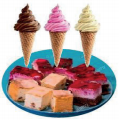We are going to have either a ice cream or a cake.
Ice cream can be selected from 3 flavors and cake from two flavors. Both the events cannot
occur simultaneously selecting ice cream and cake.
∴ Number of possible ways = 3 + 2
= 5 waysQuestion 2.
Shanthi has 5 chudithar sets and 4 frocks. In how many possible ways, can she wear either a chudithar or a frock?
Shanthi her 5 chudidhar sets and 4 frocks.
She wear either chudidhar or a frock.
∴ Total possible ways = 5 + 4 = 9 ways

Question 3.
In a Higher Secondary School, the following groups are available in XI standard
I. Science Group:
(i) Physics, Chemistry, Biology and Mathematics
(ii) Physics, Chemistry, Mathematics and Computer Science.
(iii) Physics, Chemistry, Biology and Home Science

Il. Arts Group:
(i) Accountancy, Commerce, Economics and Business Maths
(ii) Accountancy, Commerce, Economics and Computer Science
(iii) History, Geography, Economics and Commerce

III. Vocational Group:
(i) Biology, Nursing Theory, Nursing Practical I and Nursing Practical II
(ii) Home Science, Textiles and Dress Designing Theory, Textiles and Dress
Designing Practical I and Textiles and Dress Designing Practical Il
In how many possible ways, can a student choose a group?
The student either select any one of science group in 3 ways or any of the arts group in 3
ways or any of the vocational group in 2 ways.
∴ Total possible ways = 3 + 3 + 2 = 8 waysQuestion 4.
If you have 2 school bags and 3 water bottles then, in how many different ways can you choose each one of them, while going to school?
We can select one school bag from 2 and one bottle from 3 as follows.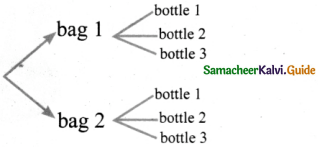∴ A bag and a water bottle can be selected in 2 × 3 = 6 ways.

Question 5.
Roll numbers are created with a letter followed by 3 digits in it, from the letters A, B, C, D and E and any 3 digits from 0 to 9. In how many possible ways can the roll numbers be generated? (except A000, B000, C000, D000 and E000)
We have a letter followed by 3 digits in the roll number.
The letter is selected from the five letters A, B, C, D, E.
For these 5 letters we have to select a 3 digit number using the digits O to 9.
Ones place can be formed using any one of the 10 number 0 to 9 in 10 ways.
Tens place can be formed in I O ways.
∴ A two digit number can be formed in 10 × 10 = 100 ways.
Thousands place can be formed in lo ways
∴ A 3 digit number can be formed in 10 × 10 × 10 = 1000 ways.
∴ 5 letters can be attached in 5 × 1000 = 5000 ways.
∴ The roll number can be formed in 5000 – 5 = 4995 ways.Question 6.
A safety locker in a jewel shop requires a 4 digit unique code. The code has the digits from 0 to 9. How many unique codes are possible?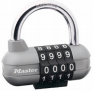The unique code has 4 digits.
Each digit is formed using any of the 10 numbers from 0 to 9.
∴ Single digit number can be formed in 10 ways.
A double digit number can be formed in 10 × 10 ways.
A three digit number can be formed in 10 × 10 × 10 ways.
A four digit number can be formed in 10 × 10 × 10 × 10 ways. = 10,000 waysQuestion 7.
An examination paper has 3 sections, each with five questions and students are instructed to answer one question from each section. In how many different ways of can the questions be answered?
The tree diagram for this may be∴ Number of possible ways to select one questions from each of 3 sections is 3 × 5 = 15 waysQuestion 8.
The given spinner is spun twice and the two numbers got are used to form a 2 digit number. How many different 2 digits numbers are possible?On the first spin we get any of the five numbers to form ones place then insecond spin the number got will fill 10’s place.
∴ Number of ways = 5 × 5 = 25 ways.
Removing the repetitions (11, 22, 33, 44, 55) once we get 25 – 5 = 20 ways.
20 different two digit numbers are possible

Question 9.
Ramya wants to paint a pattern in her living room wall with minimum budget. Help her to colour the pattern with 2 colours but make sure that no two q adjacent boxes are the same colour. The pattern is shown in the picture.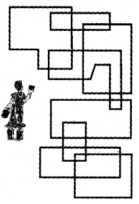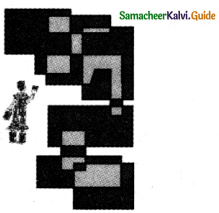Question 10.
Colour the regions in the maps with few colours as possible but make sure that no two adjacent countries are of the same colour.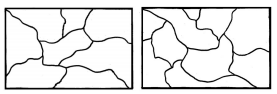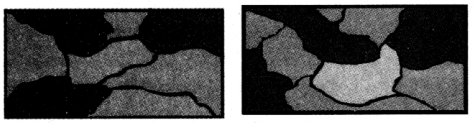Objective Type Questions

Question 11.
In a class there are 26 boys and 15 girls. The teacher wants to select a boy or a girl to represent a quiz competition. In how many ways can the teacher make this selection?
(A) 41
(B) 26
(C) 15
(D) 390
41

Question 12.
How many outcomes can you get when you toss three coins once?
(A) 6
(B) 8
(C) 3
(D) 2
8Question 13.
In how many ways can you answer 3 multiple choice questions, with the choices A,B,C and D?
(A) 4
(B) 3
(C) 12
(D) 64